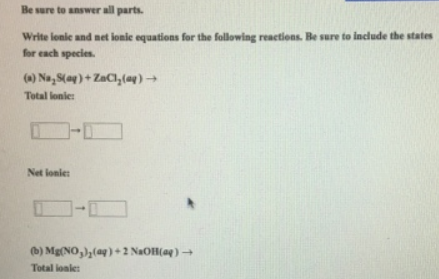# Problem: Be sure to answer all parts.Write ionic and net ionic equations for the following reactions. Be sure to include the states for each species. (a) Na2S (aq) + ZaCl2 (aq) → Total ionic: Net ionic: (b) Mg(NO3)2 (aq) + 2 NaOH (aq) → Total ionic:Net ionic:

###### FREE Expert Solution
86% (407 ratings)###### Problem Details

Be sure to answer all parts.

Write ionic and net ionic equations for the following reactions. Be sure to include the states for each species.

(a) Na2S (aq) + ZaCl2 (aq) →

Total ionic:

Net ionic:

(b) Mg(NO3)2 (aq) + 2 NaOH (aq) →

Total ionic:

Net ionic: